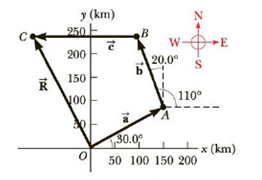Chapter 1, Problem 71P

Chapter
Section
Textbook Problem

A commuter airplane starts from an airport and takes the route shown in Figure P1.71. The plane first flies to city A, located 175 km away in a direction 30.0° north of east. Next, it flies for 150. km 20.0° west of north, to city B. Finally, the plane flies 190. km due west, to city C. Find the location of city C relative to the location of the starting point.Figure P1.71

To determine
The location of city C relative to the location of the starting point.

Explanation

Given Info: The plane flies to city A is 175km at 30°  North of East . Then the plane flies to city B is 150km at 20°  West of North . Finally, the plane flies to city C is 190km due to west.

Explanation:

The location of the cities is depicted below:

The net displacement along x direction is,

Rx=[(175km)cos(30°)]+[(150km)cos(110°)]+[(190km)cos(180°)]=89.3km

The net displacement along y direction is,

Ry=[(175km)sin(30°)]+[(150km)sin(110°)]+[(190km)sin(180°)]=229km

Formula to calculate the location of the city C from the starting point is,

R=Rx2+Ry2

• R is location of city C from starting point
• RxandRy are the x and y component of the location

Still sussing out bartleby?

Check out a sample textbook solution.

See a sample solution

The Solution to Your Study Problems

Bartleby provides explanations to thousands of textbook problems written by our experts, many with advanced degrees!

Get Started